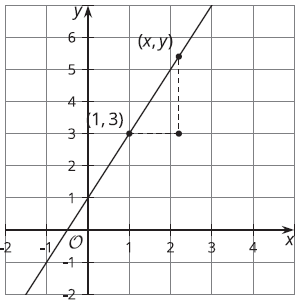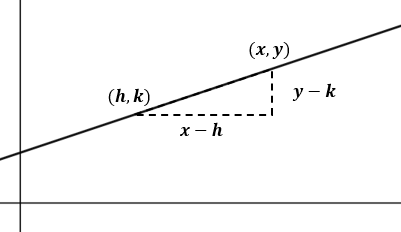# G.6.9.2 Building an Equation for a Line

The image shows a line.a. Write an equation that says the slope between the points (1, 3) and (x, y) is 2. b. Look at this equation: y - 3 = 2(x - 1). How does it relate to the equation you wrote?

Here is an equation for another line: = () a. What point do you know this line passes through? b. What is the slope of this line?

Next, let’s write a general equation that we can use for any line. Suppose we know a line passes through a particular point (h, k).a. Write an equation that says the slope between point (x, y) and (h, k) is m. b. Look at this equation: (y - k) = m(x - h). How does it relate to the equation you wrote?